### Home > A2C > Chapter 10 > Lesson 10.2.5 > Problem10-128

10-128.
1. The roots of a polynomial are given below. Find two linear factors and the corresponding quadratic factor. Homework Help ✎

1.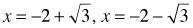2.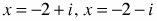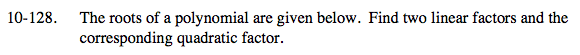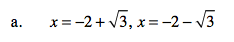Use the Zero Product Property.

$0=(x-(-2+\sqrt{3}))(x-(-2-\sqrt{3}))$

$0=(x+2-\sqrt{3})(x+2+\sqrt{3})$

y = x2 + 4x + 1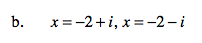Use the same process as in part (a).